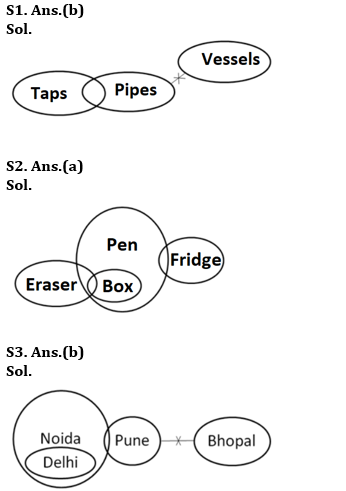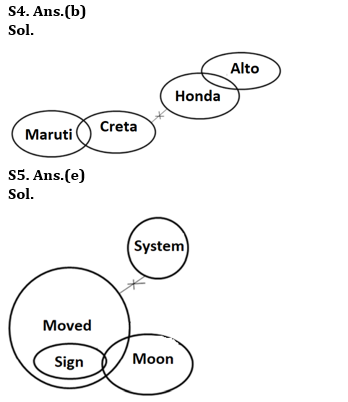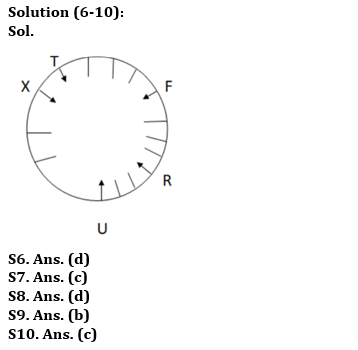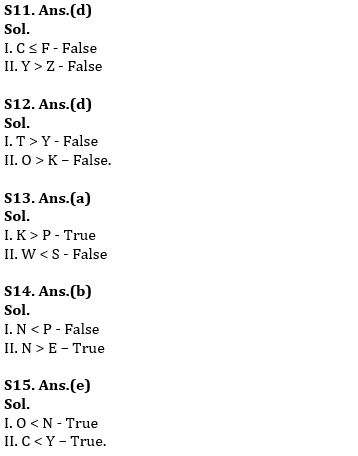Latest Banking jobs   »   RBI Assistant Vacancy 2022   »

# Reasoning Ability Quiz For RBI Assistant Prelims 2022- 18th March

Directions (1-5): In each of the questions below are given few statements followed by two conclusions numbered I and II. You have to take the given statements to be true even if they seem to be at variance with commonly known facts. Read all the conclusions and then decide which of the given conclusions logically follows from the given statements, disregarding commonly known facts.

Q1. Statements: Only a few Taps are Pipes. No Pipes is Vessels.
Conclusions:
I. Some Taps are Vessels.
II. All Taps can never be Vessels.
(a) Only I follows
(b) Only II follows
(c) Either I or II follows
(d) Neither I nor II follows
(e) Both I and II follow

Q2. Statements: Only a few Eraser are Box. All Box are Pen. Some Pen are Fridge.
Conclusions:
I. All Pens being Eraser is a possibility.
II. Some Boxes are Fridge.
(a) Only I follows
(b) Only II follows
(c) Either I or II follows
(d) Neither I nor II follows
(e) Both I and II follow

Q3. Statements: All Delhi is Noida. Some Noida is Pune. No Pune is Bhopal.
Conclusions:
I. Some Delhi is Pune.
II. Some Bhopal being Noida is a possibility.
(a) Only I follows
(b) Only II follows
(c) Either I or II follows
(d) Neither I nor II follows
(e) Both I and II follow

Q4. Statements: Some Maruti are Creta. No Creta is Honda. Only a few Honda are Alto.
Conclusions:
I. All Honda are Alto.
II. Some Maruti are not Honda.
(a) Only I follows
(b) Only II follows
(c) Either I or II follows
(d) Neither I nor II follows
(e) Both I and II follow

Q5. Statements: Only a few moon are sign. All signs are moved. No system is moved.
Conclusions:
I. No sign is system.
II. Some moon are definitely not signs.
(a) Only I follows
(b) Only II follows
(c) Either I or II follows
(d) Neither I nor II follows
(e) Both I and II follow

Directions (6-10): Study the following data carefully and answer the questions accordingly.
A certain number of people are sitting around a circular table facing the center. Two people are sitting between X and U. X is an immediate neighbor of T. F sits fourth to the right of R. More than two people are sitting between F and X. Three people are sitting between T and F. Two people are sitting between U and R.

Q6. How many people are sitting in this arrangement?
(a) 12
(b) 19
(c) 16
(d) 15
(e) None of these

Q7. How many people are sitting between X and R, when counted right of X?
(a) Four
(b) Three
(c) Five
(d) Two
(e) None of these

Q8. Which of the following is true regarding R?
(a) R is an immediate neighbor of T
(b) Five people are sitting between R and X when counted right of R
(c) U sits third to the right of R
(d) R sits third to the right of U
(e) None is true

Q9. Who are the immediate neighbors?
(a) U, X
(b) X, T
(c) F, U
(d) R, T
(e) None of these

Q10. What is the position of U from T?
(a) Third to the right
(b) Eighth to the left
(c) Fourth to the right
(d) Ninth to the left
(e)None of these

Directions (11-15): In the following questions, a statement or statements are given followed by some conclusions. Study the following data carefully and answer the questions accordingly.

Q11. Statements: W ≥ Z = C > L ≥ E ≥ S = F ≤ A, L < H ≤ Y
Conclusions:
I. C ≤ F
II. Y > Z
(a) Only I follows
(b) Only II follows
(c) Either I or II follows
(d) Neither I nor II follows
(e) Both I and II follow

Q12. Statements: O > C < Z ≥ Q > Y, T = H > K > C
Conclusions:
I. T > Y
II. O > K
(a) Only I follows
(b) Only II follows
(c) Either I or II follows
(d) Neither I nor II follows
(e) Both I and II follow

Q13. Statement: K > W = C ≥ W = P ≥ T < X ≤ E < S
Conclusions:
I. K > P
II. W < S
(a) Only I follows
(b) Only II follows
(c) Either I or II follows
(d) Neither I nor II follows
(e) Both I and II follow

Q14. Statements: Y < L ≥ C = E ≤ P > K ≤ Z; A > L < N
Conclusion:
I. N < P
II. N > E
(a) Only I follows
(b) Only II follows
(c) Either I or II follows
(d) Neither I nor II follows
(e) Both I and II follow

Q15. Statement: V > O < D < C ≤ N < K ≤ Y > A ≥ B > P
Conclusion:
I. O < N
II. C < Y

(a) Only I follows
(b) Only II follows
(c) Either I or II follows
(d) Neither I nor II follows
(e) Both I and II follow

Solutions